# Crack IBPS PO : data interpretation : Aptitude day 59

D.1-5) Automobiles produced by Tata Motors for a period of five years and its exports to various countries as given below.

Automobiles produced and stock in thousands is shown below:-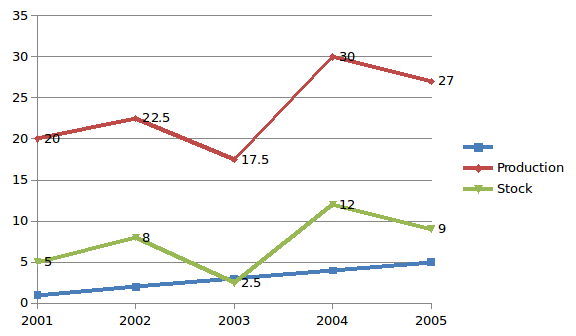Export done by Tata Motors to various countries in percentage:-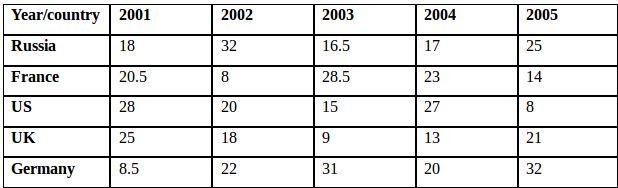1) What is the total number of exports to France, from 2001 to 2005?

A) 15,270

B) 17,170

C) 15,170

D) 20,275

E) 18,245

c)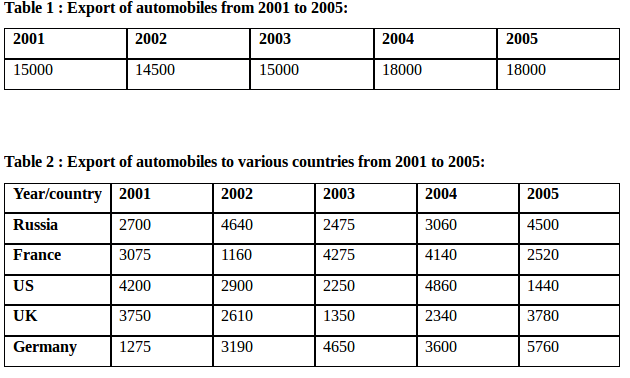From the above table 2,

Total number of exports to France = 3075+1160+4275+4140+2520 = 15,170.

2) In 2006 all the five countries reduced import of Tata Motors by 25% comparing to previous year. So by how much percentage the company must reduce its production in 2006 comparing to previous year. So, the company can maintain the same stock in 2005 & 2006.

A) 20 2/3

B) 18 2/3

C) 22 2/3

D) 16 2/3

E) None of these

c)Total export in 2005 = 18000 (from the above table 1)

Total export in 2006 = 75% of export in 2005 = 13,500

Stock in 2005 = Stock in 2006 = 9000

Production in 2005 = 27000

Production in 2006 = 13,500 + 9000 = 22,500

Percentage of production reduced in 2006 comparing to 2005 is,

=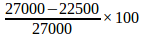= (4500/27000)*100 = 16 2/3

3) The stock in 2000 is equal to export for Germany in 2001, which is one-sixteenth of production in 2000. What percentage of export decreased in 2001, when compared to 2000?

A) 20.47%

B) 22.65%

C) 24.87%

D) 23.47%

E) 21.57%

e)Stock in 2000 = Export to Germany in 2001 = 1275 (from table 2)

Production in 2000 = 16 times of stock in 2000 = 16×1275 = 20400

Export in 2000 = 20400 -1275 = 19125

Export in 2001 = 15000 (from table 1)

Percentage of export decreased in 2001 when compared to 2000,

=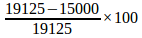=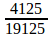*100 = 21.5686% = 21.57%

4) What percentage of export to UK in 2003 when compared to previous year? (Find the Approximate value).

A) 50 %

B) 52 %

C) 54%

D) 56%

E) None of these.

B)Export to UK in 2002 = 2610 (from table 2)

Export to UK in 2003 = 1350 (from table 2)

Percentage of export to UK in 2003 when compared to 2002 is,

=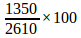= 51.72 % = 52 %.

5) What is the ratio between export to US in 2001 & 2003 to export to Russia in 2002 & 2004?

A) 129 : 154

B) 154 : 129

C) 125 : 137

D) 229 : 154

E) None of these.

A)Export to US in 2001 & 2003 = 4200 + 2250 = 6450

Export to Russia in 2002 & 2004 = 4640 + 3060 = 7700

Export to US in 2001 & 2003: Export to Russia in 2002 & 2004

6450: 7700

129:154

D.6-10) The graph below shows the number of article purchased and marked price of each article.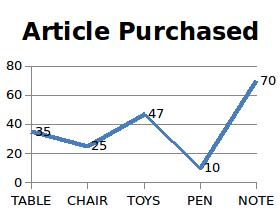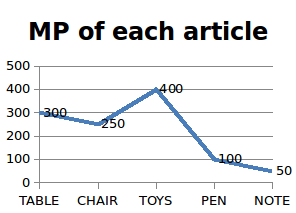6) If CP of 5 Pens is equal to CP of 10 Notes. If the man makes a profit of Rs.350 after selling all the notes by giving 10% discount on each note. Find the CP of 7 pens?

A) Rs 400

B) Rs 800

C) Rs 80

D) Rs 560

E) Rs 360

(D)

SP of 70 notes = 70×50×90/100 = Rs. 3150

CP of 70 notes = 3150 – 350 = Rs. 2800

CP of 10 notes = Rs. 400 = CP of 5 pen

CP of 1 pen = Rs. 80

CP of 7 pen = 7×80 =Rs.500

7) After purchasing all the toys the man saw few toys were get damaged. So he decided to sell the damaged toys by giving two successive discounts of 15% & 25% and remaining toys at the MP itself. After selling all the toys there is neither a profit nor loss occurs. Find the number of toys that are not damaged if CP of each toy is Rs.310.

A) 18

B) 16

C) 11

D) 10

E) None of these

C)

Let number of damaged toys be X

There is neither a profit nor a loss occurs,

So, CP of 29 toys = SP of 29 toys

29×310 = X×400×85/100*75/100 + (29-X)×400

8990 = 255X + 11600 – 400X

145X = 2610

X = 18

Number of toys that are not damaged = 29 – 18 = 11

8) A man went for shopping to buy 20 chairs with Rs. X with him but he was offered only Y (i.e. Y<20) chairs with the MP for the amount what he had. So after bargaining he was offered 20% discount on each chairs so that he can able to buy 20 chairs. Find the value of Y ?

A) 18

B) 14

C) 15

D) 16

E) 12

(D)

SP of one chair = 250×80/100 = Rs. 200

SP of 20 chairs = 20 × 200 = Rs.4000 = the amount he has initially (i.e. X)

For Rs. 4000, number of chairs offered to him (i.e. Y) with SP as MP is,

4000/250 = 16

9) If CP of 6 Table is equal to SP of 5 Table. Then the profit earned by seller on selling all 35 tables is Rs.1, 260. Find the discount offered by the seller on tables?

A) 18%

B) 28%

C) 20%

D) 26%

E) Cannot be determined.

(B)

CP of 6 Table = SP of 5 Table

Profit % =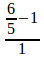×100 = 20% = Rs. 1260

SP of 35 Tables = 120% of profit =(1260/20)*120= Rs. 7560

MP of 35 Tables = 35 ×300 = Rs. 10500

Discount % = (MP – SP ) / MP*100=(10500-7560)/10500*100=28%

10) Find the ratio between SP of 22 toys with two successive discounts of 37% & 10% and SP of 33 notes with two successive discounts of 20% & 16%?

A) 10 : 2

B) 2 : 5

C) 9 : 2

D) 3 : 8

E) None of these